Ex 4.1

Chapter 4 Class 6 Basic Geometrical Ideas
Serial order wise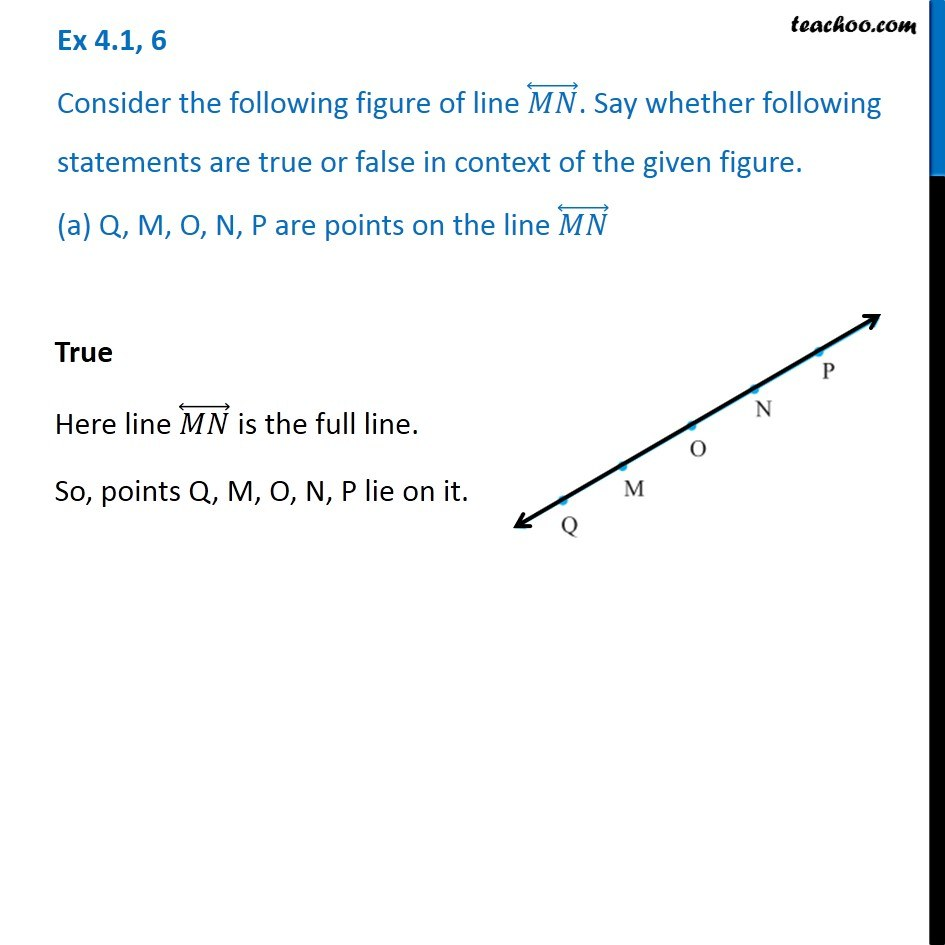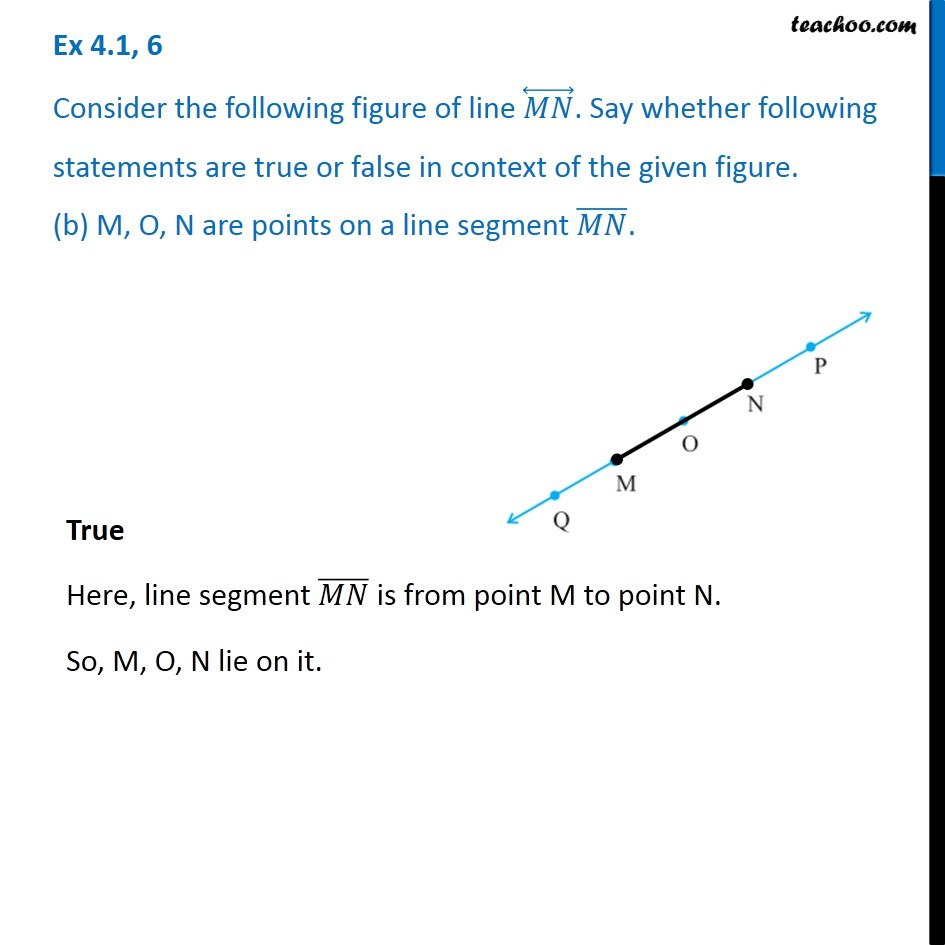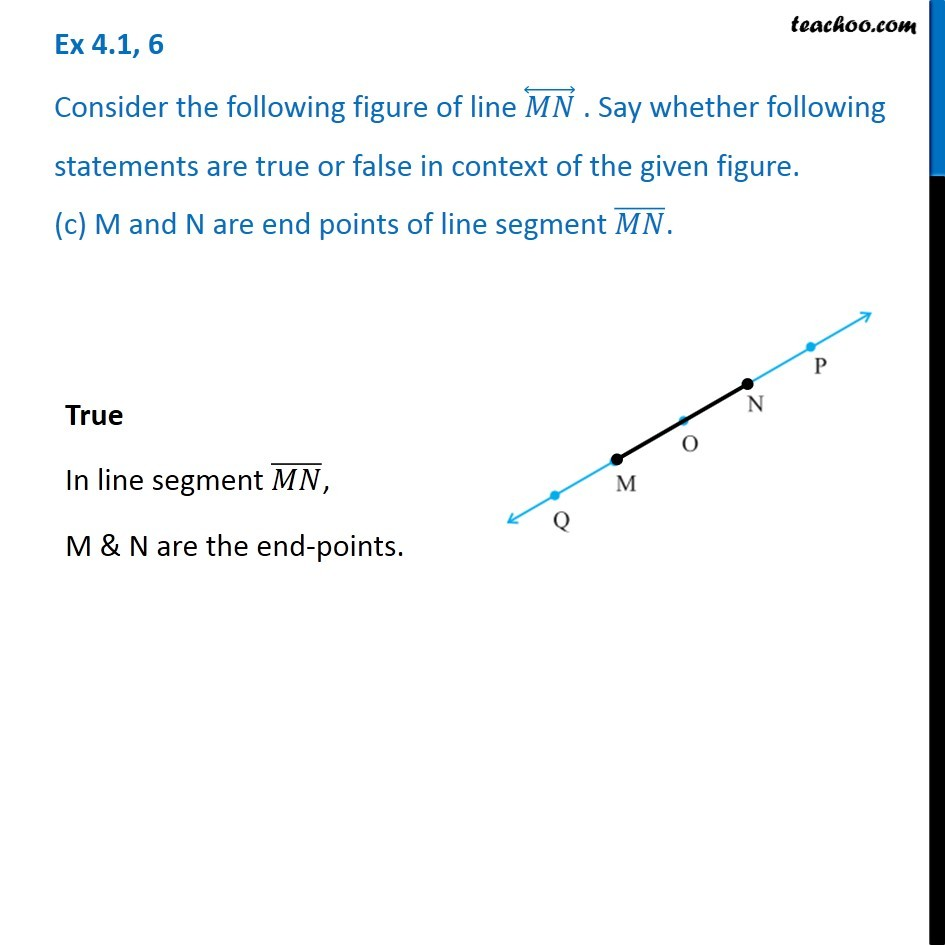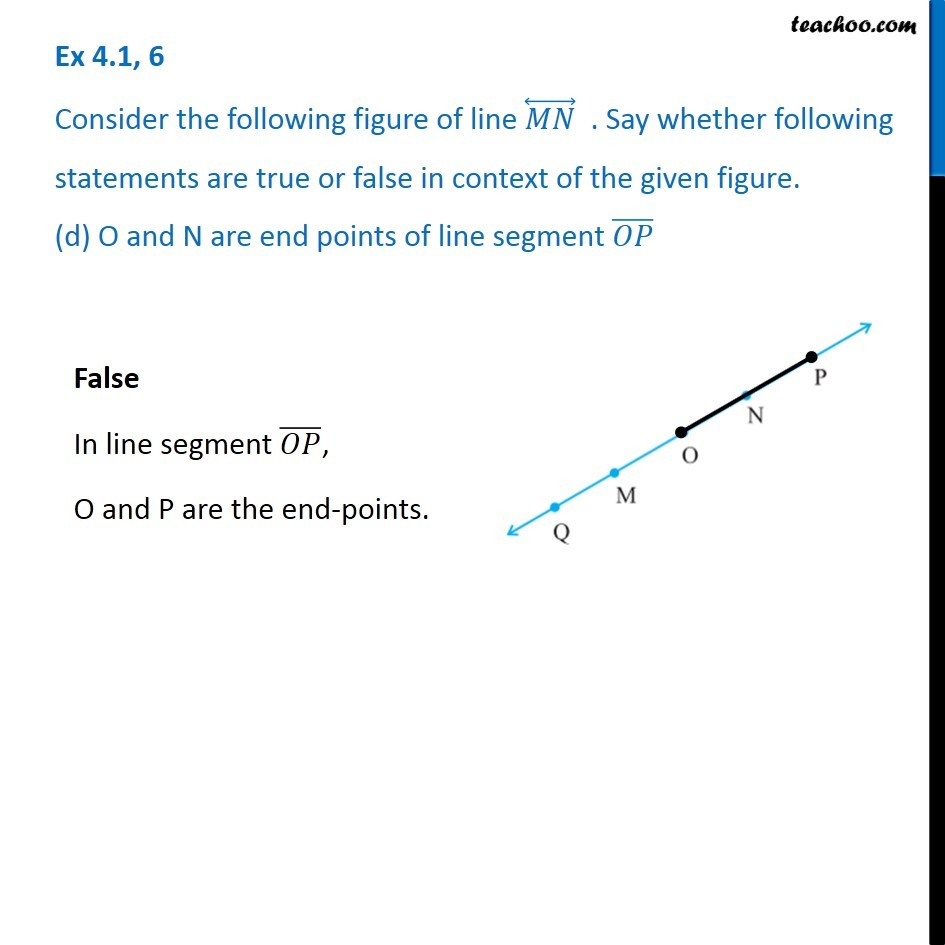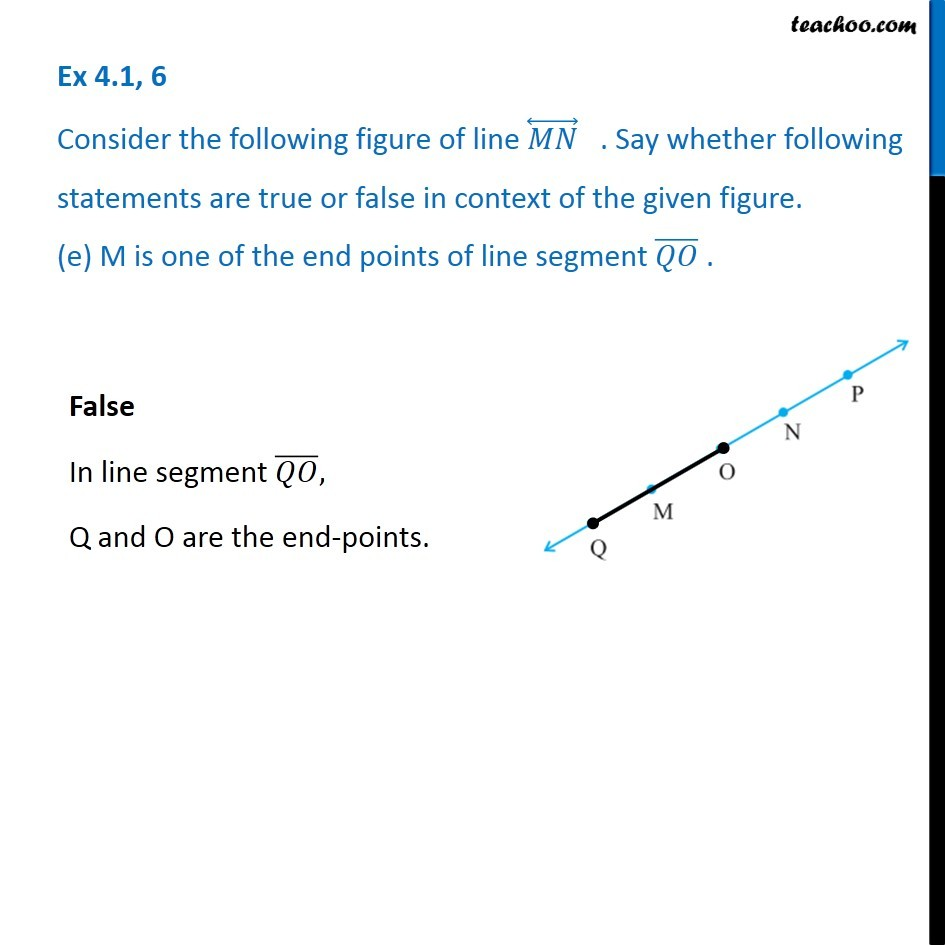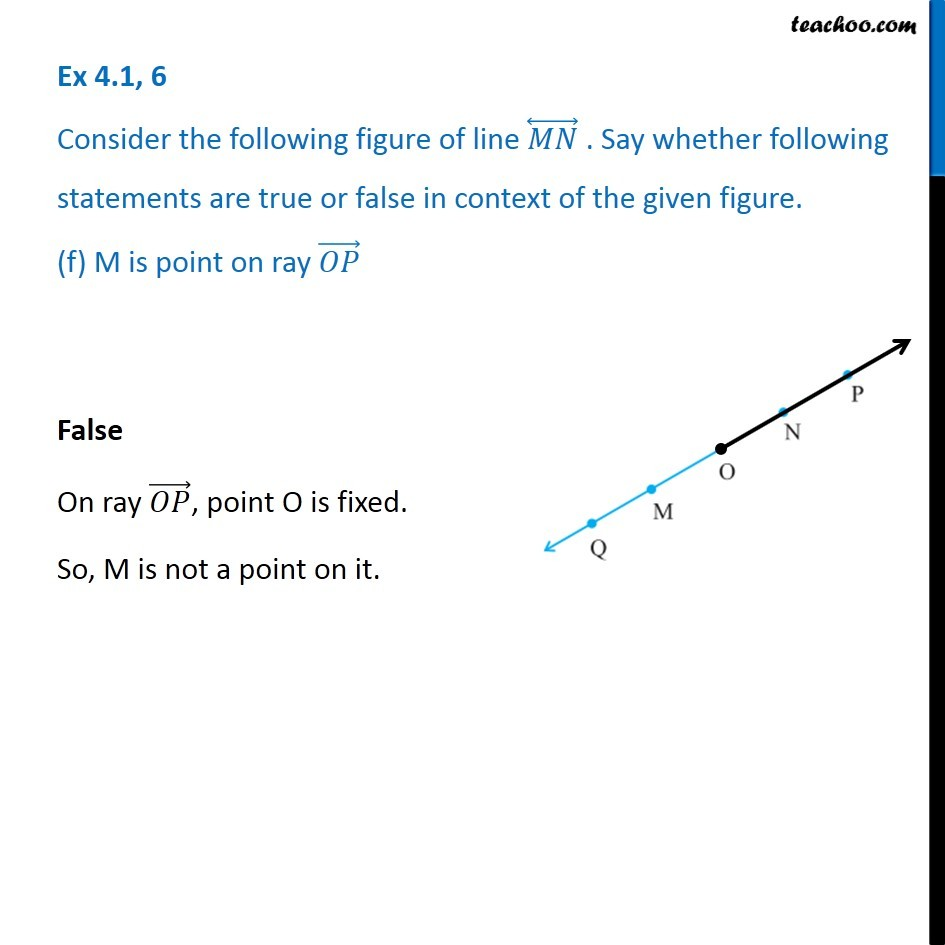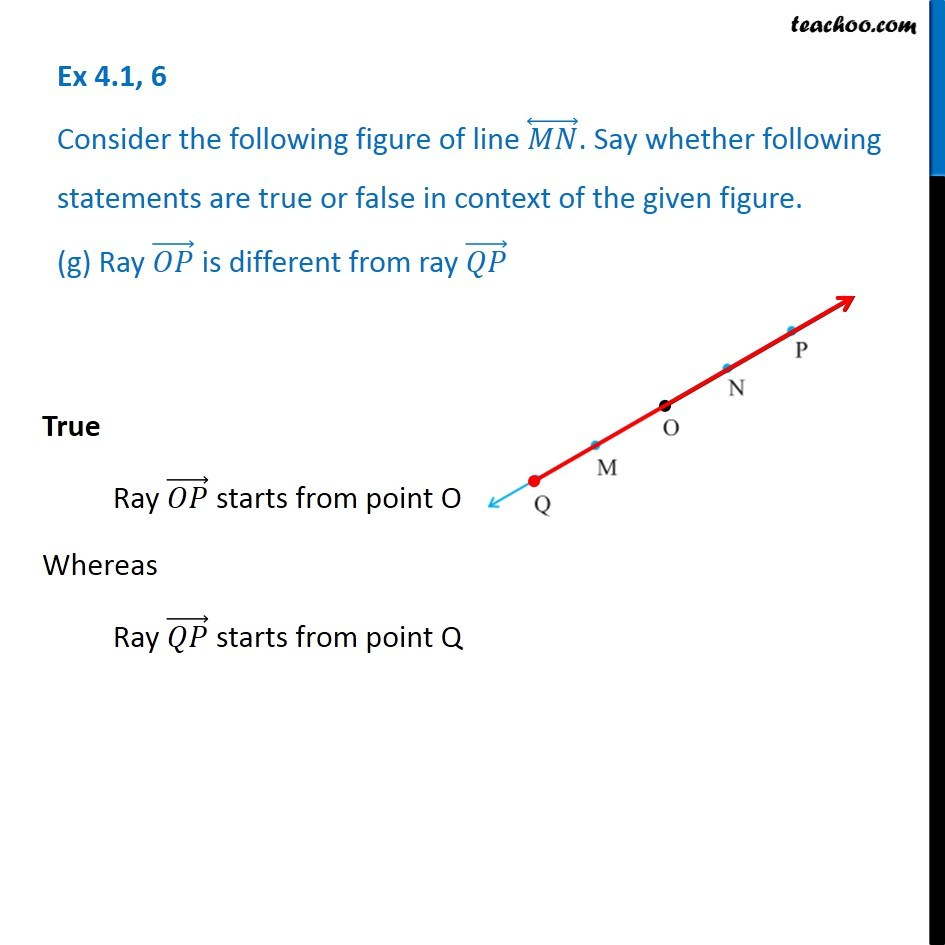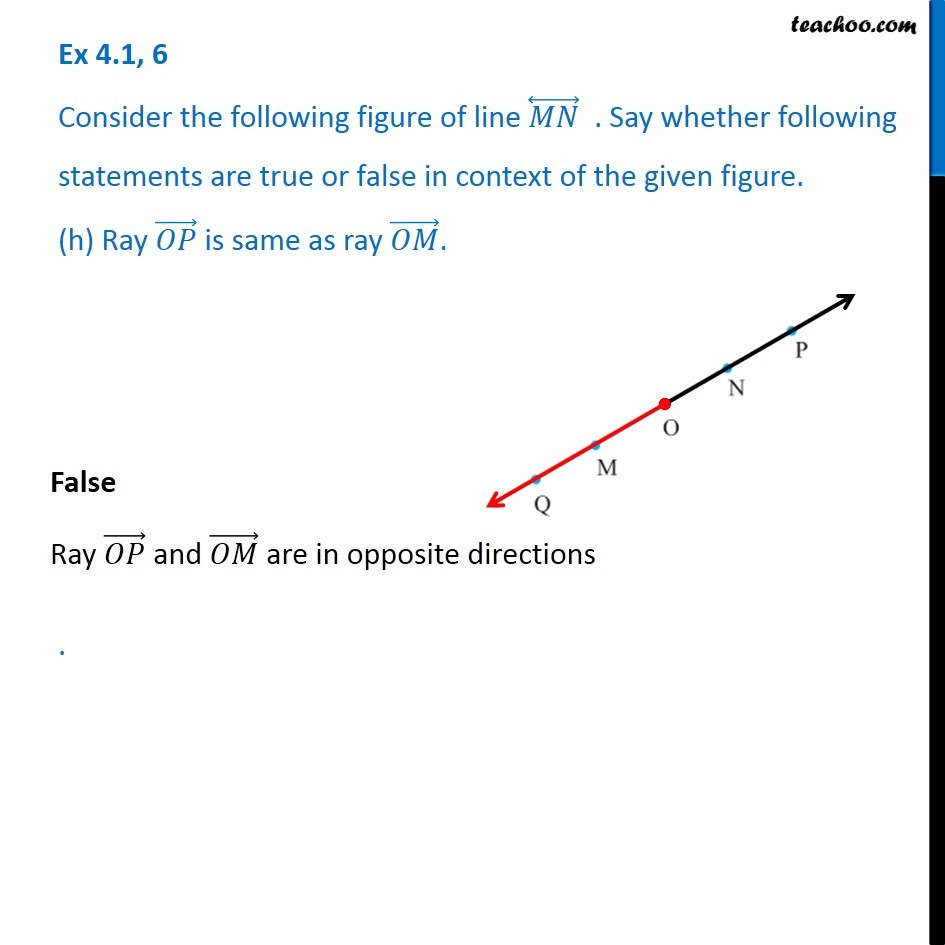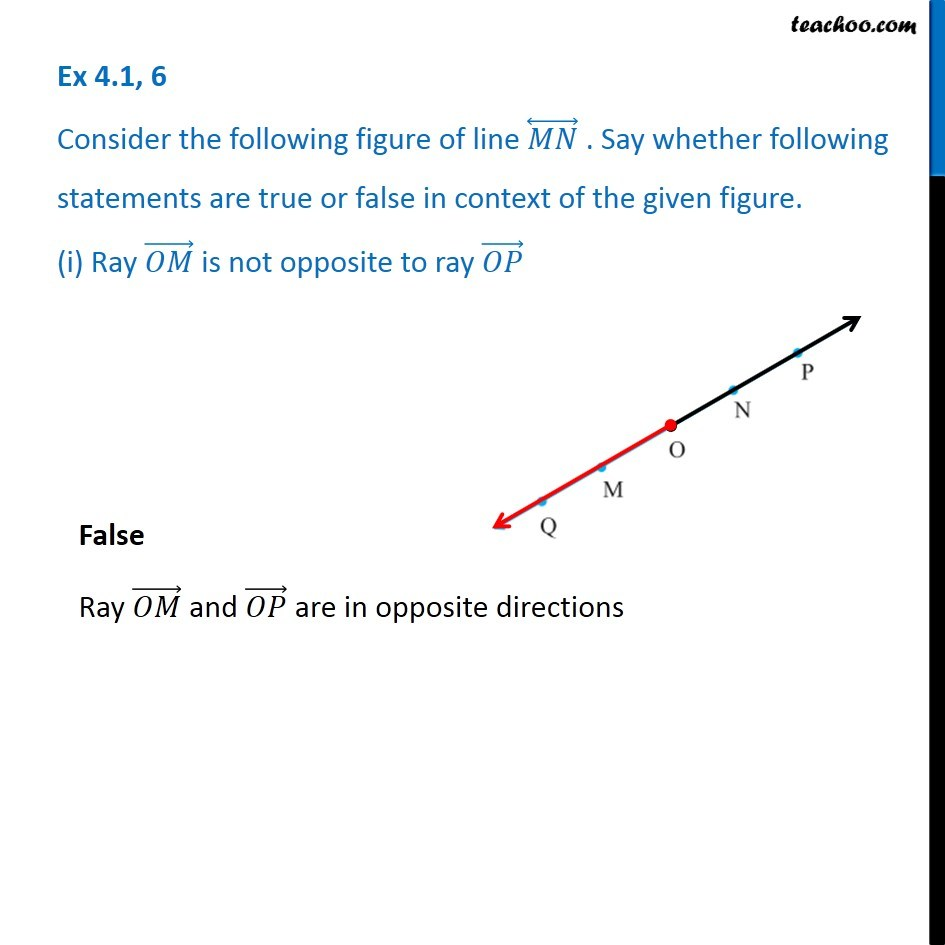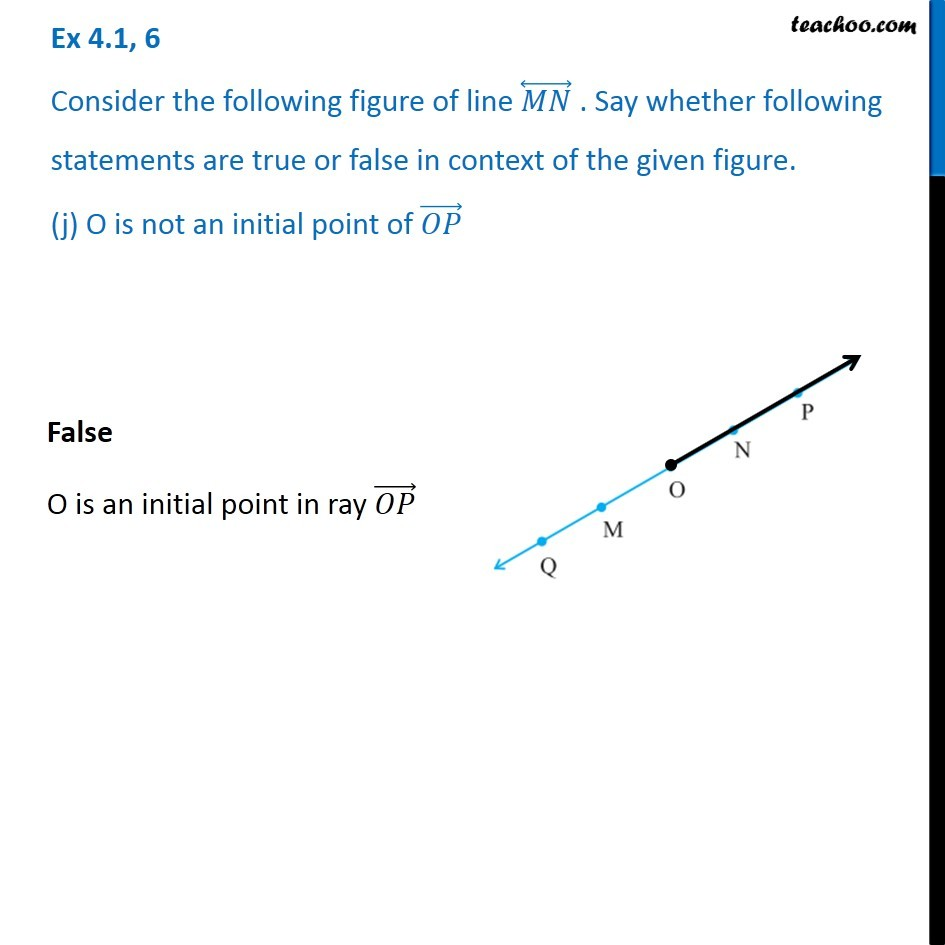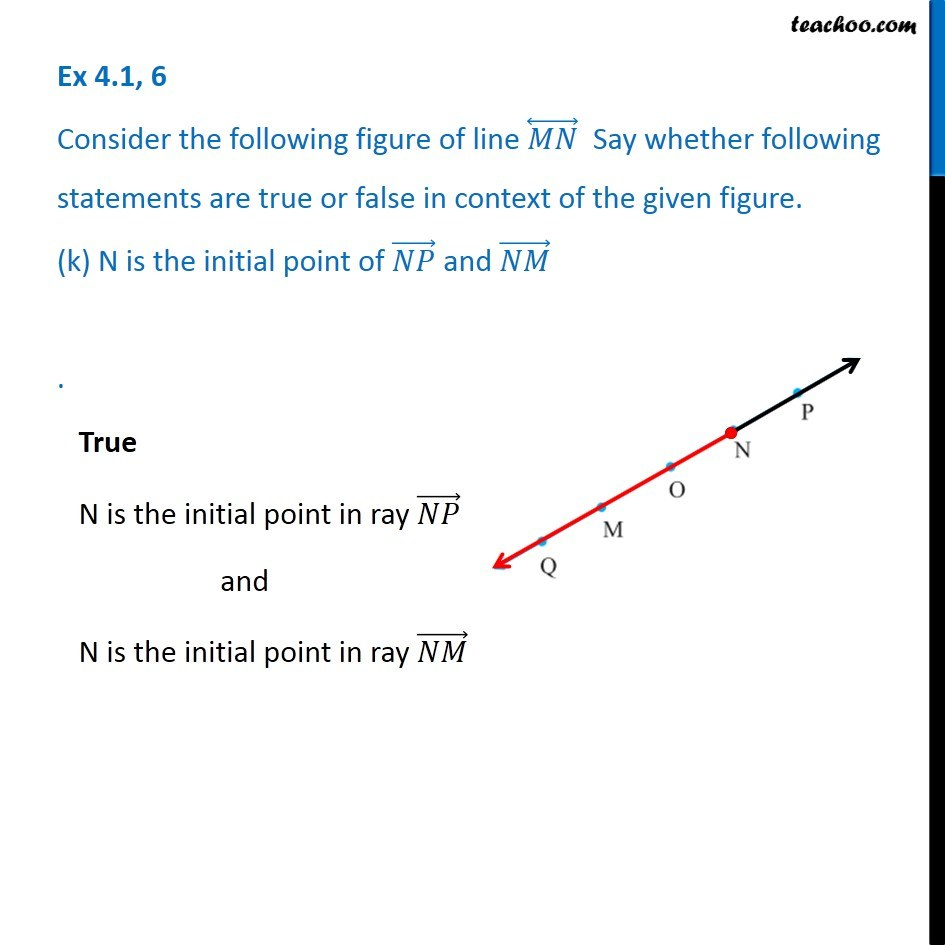Learn in your speed, with individual attention - Teachoo Maths 1-on-1 Class

### Transcript

Ex 4.1, 6 Consider the following figure of line (𝑀𝑁) ⃡. Say whether following statements are true or false in context of the given figure. (a) Q, M, O, N, P are points on the line (𝑀𝑁) ⃡ True Here line (𝑀𝑁) ⃡ is the full line. So, points Q, M, O, N, P lie on it. Ex 4.1, 6 Consider the following figure of line (𝑀𝑁) ⃡. Say whether following statements are true or false in context of the given figure. (b) M, O, N are points on a line segment (𝑀𝑁) ̅. True Here, line segment (𝑀𝑁) ̅ is from point M to point N. So, M, O, N lie on it. Ex 4.1, 6 Consider the following figure of line (𝑀𝑁) ⃡ . Say whether following statements are true or false in context of the given figure. (c) M and N are end points of line segment (𝑀𝑁) ̅.True In line segment (𝑀𝑁) ̅, M & N are the end-points. Ex 4.1, 6 Consider the following figure of line (𝑀𝑁) ⃡ . Say whether following statements are true or false in context of the given figure. (d) O and N are end points of line segment (𝑂𝑃) ̅ False In line segment (𝑂𝑃) ̅, O and P are the end-points. Ex 4.1, 6 Consider the following figure of line (𝑀𝑁) ⃡ . Say whether following statements are true or false in context of the given figure. (e) M is one of the end points of line segment (𝑄𝑂) ̅ . False In line segment (𝑄𝑂) ̅, Q and O are the end-points. Ex 4.1, 6 Consider the following figure of line (𝑀𝑁) ⃡ . Say whether following statements are true or false in context of the given figure. (f) M is point on ray (𝑂𝑃) ⃗ False On ray (𝑂𝑃) ⃗, point O is fixed. So, M is not a point on it. Ex 4.1, 6 Consider the following figure of line (𝑀𝑁) ⃡. Say whether following statements are true or false in context of the given figure. (g) Ray (𝑂𝑃) ⃗ is different from ray (𝑄𝑃) ⃗ True Ray (𝑂𝑃) ⃗ starts from point O Whereas Ray (𝑄𝑃) ⃗ starts from point Q Ex 4.1, 6 Consider the following figure of line (𝑀𝑁) ⃡ . Say whether following statements are true or false in context of the given figure. (h) Ray (𝑂𝑃) ⃗ is same as ray (𝑂𝑀) ⃗. . False Ray (𝑂𝑃) ⃗ and (𝑂𝑀) ⃗ are in opposite directions Ex 4.1, 6 Consider the following figure of line (𝑀𝑁) ⃡ . Say whether following statements are true or false in context of the given figure. (i) Ray (𝑂𝑀) ⃗ is not opposite to ray (𝑂𝑃) ⃗ False Ray (𝑂𝑀) ⃗ and (𝑂𝑃) ⃗ are in opposite directions False Ray (𝑂𝑀) ⃗ and (𝑂𝑃) ⃗ are in opposite directions False O is an initial point in ray (𝑂𝑃) ⃗ Ex 4.1, 6 Consider the following figure of line (𝑀𝑁) ⃡ Say whether following statements are true or false in context of the given figure. (k) N is the initial point of (𝑁𝑃) ⃗ and (𝑁𝑀) ⃗ . Ex 4.1, 6 Consider the following figure of line (𝑀𝑁) ⃡ Say whether following statements are true or false in context of the given figure. (k) N is the initial point of (𝑁𝑃) ⃗ and (𝑁𝑀) ⃗ . Ex 4.1, 6 Consider the following figure of line (𝑀𝑁) ⃡ Say whether following statements are true or false in context of the given figure. (k) N is the initial point of (𝑁𝑃) ⃗ and (𝑁𝑀) ⃗ . True N is the initial point in ray (𝑁𝑃) ⃗ and N is the initial point in ray (𝑁𝑀) ⃗ Ex 4.1, 6 Consider the following figure of line (𝑀𝑁) ⃡ Say whether following statements are true or false in context of the given figure. (k) N is the initial point of (𝑁𝑃) ⃗ and (𝑁𝑀) ⃗ . True N is the initial point in ray (𝑁𝑃) ⃗ and N is the initial point in ray (𝑁𝑀) ⃗